HPS 0410 Einstein for Everyone

Back to main course page

# E=mc2

John D. Norton
Department of History and Philosophy of Science
University of Pittsburgh

Einstein's famous equation has grown into one of the great symbols of the 20th century. It is the one equation in science that people recognize, if any is. It has a kind of iconic status and dual connotations: the brilliance and insight of Einstein and the darkness of atomic bombs. Images.

## Kinematics and Dynamics

So far we have looked at kinematics, the study of motions in space and time, and we have seen how Einstein's special theory of relativity has affected it.

 In kinematics, all our analyses are given solely in terms of the quantities space and time and the other quantities that can derived from them. A familiar problem is to describe when (at what time) a moving body is where (in space). In kinematics we learned from Kepler that the motion of planets is along elliptical orbits. We learned from Galileo that the trajectories of bodies in free motion above the earth's surface is along parabolas. We can also talk of speeds and velocities (=speed in a particular direction) since speeds can be derived from spaces and times. Once you know when something is where, you can determine how fast it is going. One of the major discoveries of kinematics is that, for the same initial speed, the longest trajectory results when the motion is initially elevated at 45 degrees to the horizontal.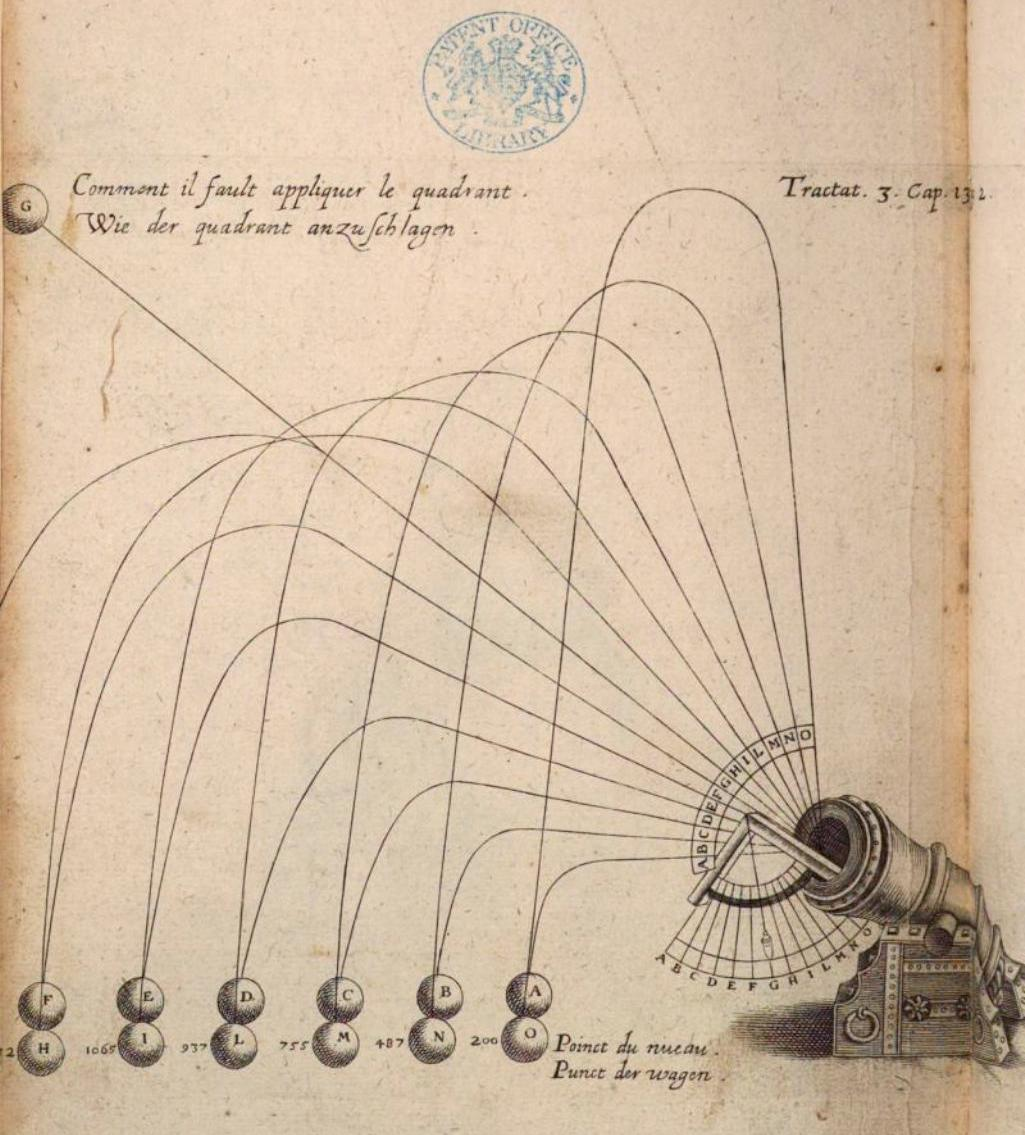Norton, The Gunner Shewing the Whole Practise of Artillery. London 1628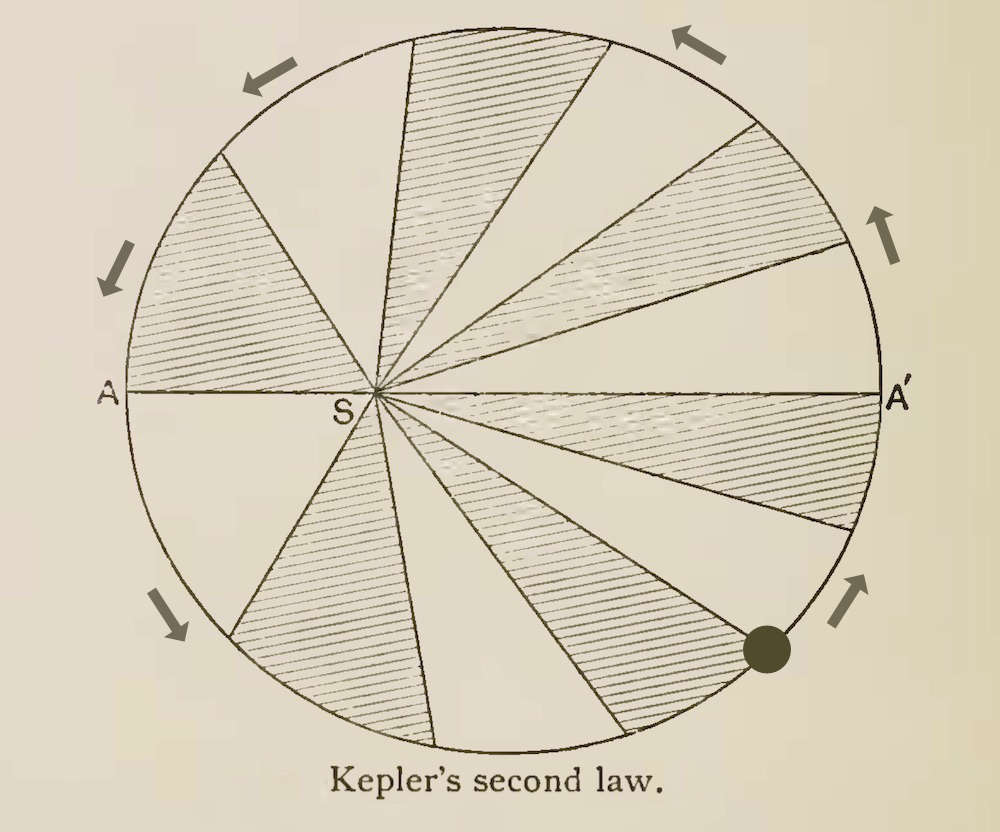Berry History of Astronomy 1898 p. 186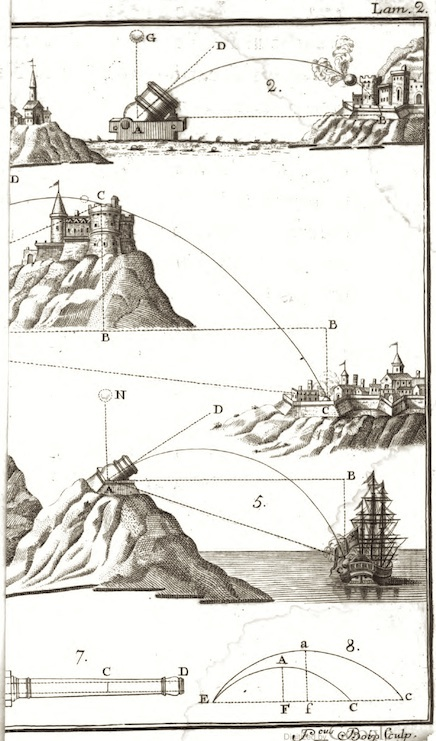We now look at dynamics, the study of the causes that affect motion, where those causes are forces. E=mc2 arises as part of the modification to dynamics brought about by Einstein's theory.

 In dynamics we expand our analyses to include new quantities associated with the causes of motion. The most important is force and two quantities derived from it, energy and momentum. We no longer just note that a tennis balls moves in a parabolic trajectory over the net. We also ask how hard we have to hit it--how much force we must apply--to send it along the desired trajectory. For the case of a cannonball, we now also ask how much gunpowder we need to load into the cannon so that the force of its explosion will send it on the desired trajectory. We want to know the energy and momentum imparted to the cannonball. It is the delivery of that energy and momentum to the target that wreaks its destructive effect.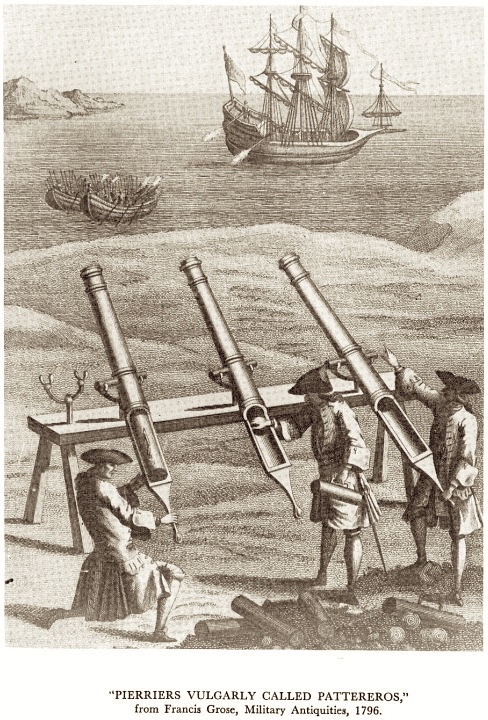In kinematics, special relativity changes our normal expectations. We find that the timing of events and distances and times elapsed are judged differently by observers in relative motion. Most relevant for the present topic, we find that we cannot accelerate anything through the speed of light. We have to adjust our ideas about space and time to accommodate this result. Since all these motions have causes treated in dynamics, we must make corresponding changes in our theories of these causes. When we make these changes in dynamics, E=mc2 results. We shall now see how that comes about.

## The Basic Concepts of Dynamics: Two Relations

Classical physics and special relativity agree in the following framework of basic concepts. That is, they both employ the concepts of energy, momentum and force and they both respect the two relations stated below that obtain between them.

Energy: I know of no useful definition for energy. It is understood by example. When systems interact, they exchange energy. For example, moving car has a certain energy of motion that is converted to heat energy in the brakes when the car is slowed. That energy originally came from chemical energy stored in the gasoline fuel, which in turn was supplied as light energy to the plants that became petroleum.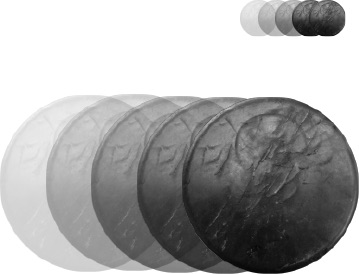Momentum: The momentum of a moving body is a measure of the quantity of motion. It is defined by      momentum = mass x velocity for a mass moving at the nominated velocity. The formula contains both mass and velocity since the quantity of the motion increases with both. (Which is the greater motion: an ounce of lead moving at 100mph or a pound of lead moving at 100mph?)

Force: When two bodies interact, the force measures the rate of transfer of momentum and energy, such as through the two relations below. That is, it measures the intensity of an interaction. It is roughly equivalent to the pre-scientific notion of muscular effort. A push can set a heavy cart in motion because it applies a force to it. Its size is given by the rate of transfer of energy and momentum.

If a constant force acts on a body, force, energy and momentum are related by the simple relations:

Momentum gained by body = Force x Time during which force acts

Energy gained by body = Force x Distance through which force acts

These relations obtain in both classical and relativistic physics. We shall see, however, that in the relativist context they turn into E=mc2.

## Conservation of Energy and Momentum

The most important laws in dynamics are those that state the conservation of energy and of momentum. These two laws can be applied whenever we have a closed system; that is, a system that does not interact with its surroundings. They assert that for such systems and any process they may undergo:

 Total Energy at start = Total Energy at end Total Momentum at start = Total Momentum at end

An isolated spaceship in deep space is a good example to consider. Imagine some interaction that takes place within that system. These laws tell us that the total of energy before the interaction equals the total of energy afterwards; and the total of momentum before the interaction equals the total of momentum afterwards.Let's look at an example of such a process. A spacewalker stands on the spaceship and both are at rest. They have no velocity, so their total momentum is zero. Momentum =mass x velocity =mass x 0 = 0

Now imagine that the spacewalker vigorously pushes off from the spaceship and floats off into space. The spacewalker has gained some momentum. If the spacewalker has mass of 200 pounds and moves off at 10 feet per second, he has gained 2,000 units of momentum. The law of conservation of momentum demands that the total momentum of the two systems stays constant. That is the total momentum of the spaceship plus spacewalker system must remain zero.That can only happen if the spaceship gains a negative momentum exactly opposite to the momentum gained by the spacewalker. That is a momentum of -2,000 units. Then the sum of the two will be zero.
-2,000 + 2,000 = 0

 And that will only happen if the spaceship gains a velocity in the direction exactly opposite to that of the spacewalker's motion; that is, if the spaceship recoils. So conservation of momentum demands a recoil. If you want to keep doing the sums, we can figure out just how big the velocity of recoil must be. If the spaceship has a mass of 2,000 lb, then the recoil is just -1ft/sec. For then its momentum is        2,000x(-1) = -2,000 ft lb/sec

Analogous considerations apply to the combined energy of the spaceship and spacewalker. After the interaction both spaceship and spacewalker have some energy of motion. That energy originated as chemical energy stored in the muscles of the spacewalker, before the spacewalker used muscle power to push off the spaceship. The energy of motion gained by the system must match the chemical energy lost from the spacewalker's muscles so that the total energy stays constant.

## Achieving Unlimited Velocities in Classical Physics

Unlike relativity theory, classical physics allows us to accelerate bodies to arbitrarily high speeds. There is a simple mechanism for achieving these unlimited speeds in classical physics. If we keep applying a constant force to a body, the body will keep gaining energy and momentum and its velocity will rise accordingly.How can we go about applying a constant force to a body over a long enough time period to achieve very high velocities? If the body is very small, it turns out to be much easier than you might imagine. If the body is a very small particle--an electron say--then the forces of electric and magnetic fields can quite quickly accelerate the particles to close to the speed of light. Over a hundred years ago, this happened in the first cathode ray tubes, that is, in devices like old fashioned TV tubes. If a high voltage is applied between two metal plates in a near evacuated vessel, the electric field resulting from the voltage is quite capable of pulling electrons off one electrode at very high speeds. This same technique is still used today in particle accelerators in which various particles are accelerated to close to the speed of light by combinations of electric and magnetic fields.

What about accelerating ordinary objects up to very high speeds by ordinary means? What about the most familiar method of all, swatting a ball with a bat or a club? You might suspect that this procedure is self-defeating. If I want to swat a ball to get it to move quickly, wouldn't I need a faster moving bat to swat it with? And once it is moving fast, wouldn't I need a yet faster moving bat to get it to move still faster? So can the method only yield high speeds if I already have something moving at even higher speeds?

These worries turn out to be misplaced. It is easy to see that a small ball at rest, hit by a much heavier bat, will be accelerated to twice the speed of the bat. This, for example, is pretty much what happens when a golf club hits a golf ball. The greatest speed the ball can achieve is twice that of the club's head. The argument that shows it is much easier than you would expect and uses the principle of relativity to make it simple. Look here for details.

Here's what we find happens when a small mass is struck by a very heavy mass moving at 100,000 miles per second. If the collision is elastic, the mass is boosted to 200,000 miles per second.It also turns out that it is quite easy to imagine systems that use repeated collisions that would accelerate bodies, according to classical physics, to arbitrarily high speeds. Here's one simple set up. We have two very massive blocks rolling towards one another on rails. A small elastic body is trapped in between, perhaps suspended by a rope from a high support. It is set in motion by a collision with one of the blocks. The body then bounces back and forth between the two approach blocks.With each bounce, according to classical physics, the body gains the same increment of speed and same increment in the magnitude of its momentum.By choosing the sizes and distances carefully, we can set things up so that there are as many collisions as we like. While the mass bounces back and forth between the approaching blocks, the collisions happen more and more rapidly and the mass goes faster and faster. According to classical physics, this arrangement is quite able to accelerate the mass past the speed of light, as long as the blocks are massive enough and the materials strong enough not to break in the violent collisions.

These schemes illustrate how it is possible, according to classical physics, to impart unlimited momentum to a body and, as a result, to boost it to unlimited velocities, including those greater than that of light.

We have seen that relativity theory prohibits boosting bodies past the speed of light. Therefore we must pay attention to how it arises in classical physics. Then we can decide how we must modify classical dynamics, so that it does not allow us to accelerate objects through the speed of light.

 Momentum = mass x velocity increases without limit fixed increases without limit

Recall that momentum is mass x velocity. Since the mass is a fixed number, characteristic of the body, if the body's momentum increases, so must its velocity. If its momentum grows without limit, then its velocity also increases without limit. As a result, classical physics tells us that we can accelerate masses through the speed of light.

## The Unlimited Momentum Loophole Closed

In relativistic physics, we can also supply unlimited momentum to a body. Indeed we can use the same mechanism as in classical physics--just set up a small object to collide with a larger one. And with successive collisions, we can supply more and more momentum to the small object. However, as we saw in the case of just one collision, these processes will never accelerate the small object past the speed of light.

Somehow we have to make sense of this prohibition on accelerating objects through the speed of light. We still have the relation momentum = mass x velocity. We can increase the momentum without limit. So why doesn't the velocity also increase without limit?

In the classical context, getting to the conclusion of unlimited velocity depended on an assumption: the mass of the object is constant. That is the only assumption we have left to adjust. That is how Einstein modified dynamics in 1905. The mass of the object increases with its velocity. Schematically:

 increases without limit increases only as far as c Momentum = mass x velocity SO... mass must increase when velocities get close to c

So as we put more and more momentum into the body, the velocity ceases to rise without limit; the mass starts to rise instead. Eventually, once the velocity has gotten close to that of light, all the increase is associated with the mass. This effect on a mass, as we repeatedly double its momentum, is shown in the table:

 Momentum Mass Velocity (in units of 1,000     miles/sec) 0 1 0 118.6 1.186 100 237.2 =118.6x2 1.621 146.4 474.4 =237.2x2 2.740 173.2 948.8 =474.4x2 5.198 182.5 1897.6 =948.8x2 10.251 185.1 3795 =1897.6x2 20.43 185.8 7590 =3795x2 40.82 185.9 ... ... ... ∞ ∞ c=186

Only for those of you who have to know, the formula used to determine the mass m is... (click).

In sum, according to relativity theory, a force--no matter how big or long acting--cannot accelerate a body through the speed of light. The closer the body gets to the speed of light, the greater its mass becomes and the harder it gets to accelerate. The mass grows without limit.

In 1905, this was not such a shocking way to view things. It was then known that when electrons moved close to the speed of light in cathode ray tubes, they got harder and harder to accelerate, as if they were becoming more massive. Prior to Einstein, this was explained as a complicated interaction between the electron and its electromagnetic field. Einstein now just said that the electron's mass wasn't merely appearing to increase; it was increasing.

For more on how relativity blocks a scheme for using collisions to boost things through the speed of light, see this account of the Resolution of Collisions in Classical and Relativistic Physics.

## Simple Redescription of the Growth of Mass

When a body is accelerated, we add momentum to it and its mass increases. We also add energy to the body. There turns out to be an especially simple rule that connects the energy added with the mass gained:

etc.

More generally:

Turning this around, we can say:

1 unit of mass --> c2 units of energy
2 unit of mass --> 2c2 units of energy
etc.

More generally:

m units of mass --> mc2 units of energy

This is Einstein's celebrated equation: E = mc2 seen in one application. It turns out that the relation can be derived in this case with very little more fuss merely by combining the two relations we saw above for energy, momentum and force. For the brave: show me.

Einstein's original 1905 demonstration of E = mc2 is only a little more complicated. A simplified version can be found here in Section 5 of "Einstein's Special Theory of Relativity and the Problems in the Electrodynamics of Moving Bodies that Led him to it." pp. 72-102 in Cambridge Companion to Einstein, M. Janssen and C. Lehner, eds., Cambridge University Press.

## E = mc2 at last

This famous equation asserts an equivalence of energy and mass. Whenever a body gains or loses mass or energy it gains or loses a corresponding amount of energy or mass according to the conversion formula E = mc2. We have seen this for mass gain under an applied force. That is, we have seen the result for one particular form of energy, energy of motion. When a body loses or gains energy of motion, it loses or gains mass according to E = mc2.

What about other forms of energy? What about heat energy, chemical energy, electrical energy, etc. Do bodies also lose and gain mass according to E = mc2 when they lose or gain these forms of energy? Yes they do, but it takes a little bit more argumentation to establish the result.

The argument that establishes this is a little complicated, so it is included here for the brave only.

We know that energy of motion has mass. The conservation of momentum requires that if this holds for one form of energy, it must hold for all. To see this, imagine that we have some conversion of energy of motion into another form of energy. For example, we are in an isolated spaceship with a rapidly spinning flywheel. The flywheel has considerable energy of motion and thus a corresponding mass. We now use the motion of the flywheel to turn an electrical generator that then charges a battery. The battery stores the electrical energy as chemical energy. The flywheel has lost some energy of motion; the battery has gained the corresponding amount of chemical energy. The flywheel has also lost some mass, according to E = mc2. Will the battery gain the corresponding amount of mass as well?

To see that it must, view the entire process from another spaceship that sees this system of flywheel and battery moving with uniform speed. The process will not alter the velocity of the flywheel plus battery system. Conservation of momentum demands that the total momentum of the flywheel plus battery remain the same. Therefore, since momentum = mass x velocity, the total mass of the system must stay the same. But that can only happen if the mass lost by the flywheel reappears as mass in the battery and it does so exactly in accord with E = mc2. The example can be repeated with a conversion of energy of motion to any other type of energy.

Einstein's 1905 demonstration mentioned above is distinctive in that it automatically shows that any form of matter conforms with E = mc2 if it is possible for the matter to emit radiation.

Put most briefly, Einstein's equation says that energy and mass are really just two different names for the same thing. They rise and fall together because they are at heart the same thing. We like to call that thing mass when it is in hard, lumpy forms like bricks. We prefer to call it energy when it is in the form of radiation. But the one is just a form of the other.

Here's a simple analogy. Think about the stuff we call H2O. When it is solid, we call it ice. When it is liquid, we call it water. When it is vaporized, we call it steam. But it is always the same stuff.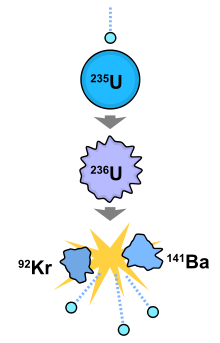http://en.wikipedia.org/wiki/File:Nuclear_fission.svg What is most important for practical purposes is that the conversion factor c2 is a huge number. That means that a small amount of mass under conversion yields a huge amount of energy. This is the principle behind nuclear weapons and nuclear power. In a nuclear fission reaction, a Uranium atom nucleus "fissions"--that is splits--into atomic nuclei of smaller size and other particles such as neutrons. It turns out that the total mass of these decay products is just slightly less than the mass of the Uranium nucleus we started with. This mass defect is around a tenth of one percent of the mass. This missing mass has been converted into energy. Because c2 is so large, the result of converting even a small part of the mass into another form is the release of a huge amount of energy. But because each Uranium nucleus is so very small, the energy released when one nucleus splits is still small.http://en.wikipedia.org/wiki/File:Nagasakibomb.jpg Now things get interesting. What triggers a Uranium atom nucleus to fission is an impact by a neutron. When the nucleus splits, it releases more neutrons, such as the two or three in the reactions shown. These neutrons can then initiate the fission of further Uranium nuclei; and the resulting neutrons initiate the fission of still further Uranium nuclei. If nothing restricts this process, we have an uncontrolled chain reaction that releases a huge amount of energy. That is an atom bomb.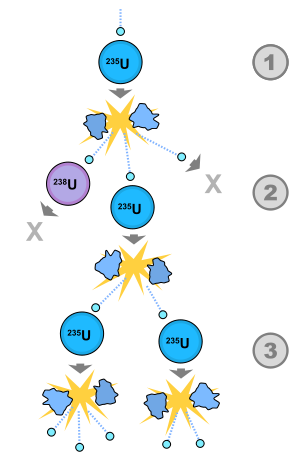http://en.wikipedia.org/wiki/File:Nuclear_fission.svg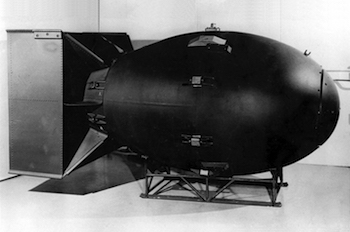http://commons.wikimedia.org/wiki/File:Fat_man.jpg

If, however, we introduce moderators that absorb the neutrons, we can slow down the chain reaction so that we have a controlled release of heat. That heat can be used to do useful things like boil water to make steam to drive electric generators. Then we have a nuclear power plant.http://commons.wikimedia.org/wiki/File:Cooling_towers_of_Dukovany_Nuclear_Power_Station.jpg

One gram of matter, about 20 drops of water, if it were fully converted into electrical energy, would be 25,000,000 kilowatt hours of electrical energy. That is enough energy to power a 100 watt light bulb for 250,000,000 hours or 28,500 years. Recorded history extends only about 12,000 years.At 5 cents a kilowatt hour, it would cost \$1,250,000 if purchased from a utility company. The energy of that same gram, if released in an explosion, would be equivalent to 21,000 tons of TNT.Image: http://en.wikipedia.org/wiki/File:Gluehlampe_01_KMJ.png
In 1905, Einstein did not expect this sort of application of his result, which then seemed to be purely of theoretical interest.